BOUNDS Statement

BOUNDS b_con [ , b_con...] ;
 where b_con := number operator parameter_list operator number or b_con := number operator parameter_list or b_con := parameter_list operator number and operator :=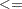, <,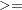, or >

Boundary constraints are specified with a BOUNDS statement. One- or two-sided boundary constraints are allowed. The list of boundary constraints are separated by commas. For example:

```bounds 0 <= a1-a9 X <= 1, -1 <= c2-c5;
bounds b1-b10 y >= 0;
```

You can specify more than one BOUNDS statement. If you specify more than one lower (upper) bound for the same parameter, the maximum (minimum) of these is taken.

If the maximum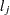of all lower bounds is larger than the minimum of all upper bounds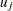for the same parameter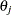, the boundary constraint is replaced by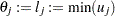defined by the minimum of all upper bounds specified for.